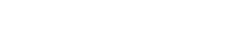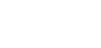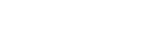Hft | Learning difficulties & other needs | Dyscalculia

## Dyscalculia

Developmental dyscalculia is sometimes described as ‘maths dyslexia’. It is a specific learning difficulty which makes learning basic arithmetic facts, processing numbers and carrying out calculations difficult.

### Signs of dyscalculia

Signs of dyscalculia include:

• A poor sense of number and estimation
• Weak mental arithmetic skills
• Difficulty in remembering mathematical facts and procedures, even with extensive practice
• Taking a very long time over calculations
• Difficulty counting backwards
• Inability to tell whether answers are right or nearly right

•••